# PID VI

LabVIEW 2012 PID and Fuzzy Logic Toolkit Help

Edition Date: June 2012

Part Number: 370401J-01

»View Product InfoDownload Help (Windows Only)

Owning Palette: PID VIs

Requires: PID and Fuzzy Logic Toolkit

Implements a PID controller using a PID algorithm for simple PID applications or high speed control applications that require an efficient algorithm. The PID algorithm features control output range limiting with integrator anti-windup and bumpless controller output for PID gain changes. Use the DBL instance of this VI to implement a single control loop. Use the DBL Array instance to implement parallel multi-loop control.

Use the pull-down menu to select an instance of this VI.

 Select an instance PID (DBL)PID (DBL Array)

## PID (DBL)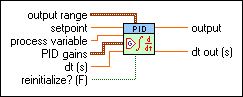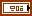output range specifies the range to which to coerce the control output. The default range is –100 to 100, which corresponds to values specified in terms of percentage of full scale. You can change this range to something that is appropriate for your control system. For example, you can relate engineering units to engineering units instead of percentage to percentage. This VI implements integrator anti-windup when the controller output is saturated at the specified minimum or maximum values.output high specifies the maximum value of the controller output. The default is 100.output low specifies the minimum value of the controller output. The default is –100.setpoint specifies the setpoint value, or desired value, of the process variable being controlled.process variable specifies the measured value of the process variable being controlled. This value is equal to the feedback value of the feedback control loop.PID gains specifies the proportional gain, integral time, and derivative time parameters of the controller.proportional gain (Kc) specifies the proportional gain of the controller. The default is 1. In the equation that defines the PID controller, KC represents the proportional gain.integral time (Ti, min) specifies the integral time in minutes. The default is 0.01.derivative time (Td, min) specifies the derivative time in minutes. The default is 0.dt (s) specifies the loop-cycle time, or interval in seconds, at which this VI is called. If dt (s) is less than or equal to zero, this VI calculates the time since it was last called using an internal timer with 1 ms resolution. If dt (s) must be less than 1 ms, specify the value explicitly. The default is -1.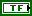reinitialize? specifies whether to reinitialize the internal parameters, such as the integrated error, of the controller. Set reinitialize? to TRUE if your application must stop and restart the control loop without restarting the entire application. The default is FALSE.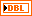output returns the control output of the PID algorithm that is applied to the controlled process. If this VI receives an invalid input, output returns NaN.dt out (s) returns the actual time interval in seconds. dt out (s) returns either the value of dt (s) or the computed interval if you set dt (s) to –1.

## PID (DBL Array)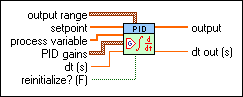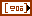output range specifies the range to which to coerce the control output. The default range is -100 to 100, which corresponds to values specified in terms of percentage of full scale. You can change this range to something that is appropriate for your control system. For example, you can relate engineering units to engineering units instead of percentage to percentage. This VI implements integrator anti-windup when the controller output is saturated at the specified minimum or maximum values.

output range is an array of clusters of the following elements.output high specifies the maximum value of the controller output. The default is 100.output low specifies the minimum value of the controller output. The default is –100.setpoint specifies the setpoint value, or desired value, of the process variable being controlled. This VI resizes the setpoint input array to match the size of the process variable input array.process variable specifies the measured value of the process variable being controlled. This value is equal to the feedback value of the feedback control loop.PID gains is an array of clusters of the following elements.proportional gain (Kc) specifies the proportional gain of the controller. The default is 1. In the equation that defines the PID controller, KC represents the proportional gain.integral time (Ti, min) specifies the integral time in minutes. The default is 0.01.derivative time (Td, min) specifies the derivative time in minutes. The default is 0.dt (s) specifies the loop-cycle time, or interval in seconds, at which this VI is called. If dt (s) is less than or equal to zero, this VI calculates the time since it was last called using an internal timer with 1 ms resolution. If dt (s) must be less than 1 ms, specify the value explicitly. The default is -1.reinitialize? specifies whether to reinitialize the internal parameters, such as the integrated error, of the controller. Set reinitialize? to TRUE if your application must stop and restart the control loop without restarting the entire application. The default is FALSE.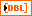output returns the control output of the PID algorithm that is applied to the controlled process. This VI determines the length of the output array from the size of the process variable input array.dt out (s) returns the actual time interval in seconds. dt out (s) returns either the value of dt (s) or the computed interval if you set dt (s) to –1.

## PID Details

You can use the DBL Array instance of this polymorphic VI in multi-loop PID control applications. In this case, the length of the process variable input determines the length of the output array. Other input arrays do not necessarily need to be the same length as the process variable input. This VI resizes other input arrays to the same length as the process variable input as follows:

• If the input array is longer than the process variable input, the input array is truncated to the length of the process variable input. Additional values in the array are not used.
• If the input array is shorter than the process variable input, the last value of the input array is repeated until the size matches that of the process variable input.

In this manner, an input value that must be used for each output calculation does not need to be specified repeatedly in the array passed into this VI. Instead, the array can consist of a single value that is used for each output calculation.

## Example

Refer to the General PID Simulator VI in the labview\examples\control\PID directory for an example of using the PID VI.